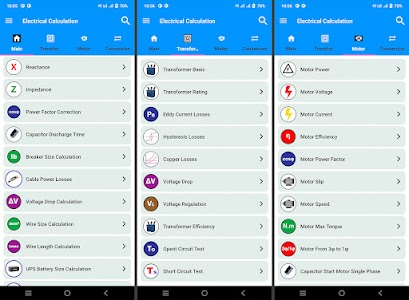# Electrical Calculation App v1

Free

### Electrical Calculation App / Screenshots### Electrical Calculation App / Description

The electrical calculation app is useful and best for electrical engineers and electricians. It has many calculations that can help you in your work. This app is very useful for the electrical engineers and electricians who work in the electric field on a daily basis.
The electrical calculation app is a very useful calculation app used to help electrical engineers make their lives easier. The electrical calculation app contains all types of information that electrical engineers require like electrical formulas for voltage current and efficiency all available in the electrical calculation app.
We can use an electrical calculator that makes sure to calculate the particular electrical quantities.
Using an electronic calculator app you can measure and analyze electrical formulas electrical resistance electric charge electrical work and electrical current.
Users can also use this electrical app as an electrical dictionary because of its ultimate uses and available features for all electricians Although the Electrical Calculations app is one of the electrical calculator apps for the electrical sector So don't miss this electrical panel load calculator app in your smartphones!

Main Calculations (Electrical formula calculator app):

• Resistor color code.
• Resistor combination.
• Inductor combination.
• Current.
• Voltage.
• Resistance.
• Active power.
• Apparent power.
• Reactive power.
• Power factor.
• Antenna length.
• Voltage divider.
• Current divider.
• Capacitive voltage divider.
• Inductive voltage divider.
• Joules effects.
• Reactance.
• Impedance.
• Power factor correction.
• Capacitor discharge time.
• Breaker size.
• Cable power loss.
• Voltage drop.
• Wire size.
• Wire length.
• Battery size.
• LC Resonance.
• Current density.
• Electrical energy.
• Capacitance to reduce voltage.
• Copper wire self-inductance.
• Air core flat spiral inductance.
• Grounding strap inductance.
• Parallel wire impedance.

• Transformer Calculations:
• Transformer basic.
• Transformer rating.
• Eddy's current losses.
• Hysteresis losses.
• Copper losses.
• Voltage drop in the transformer.
• Voltage regulation in a transformer.
• Transformer efficiency.
• Open circuit test.
• Short circuit test.

• Motor Calculation:
• Motor power.
• Motor voltage.
• Motor current.
• Motor efficiency.
• Motor power factor.
• Motor slip.
• Motor speed.
• Motor Max torque.
• Motor from three-phase to single phase.
• Capacitor starts a single motor phase.
• Motor starting time.
• Motor power of the fan.

Conversions:
• Current conversion.
• Voltage conversion.
• Temperature conversion.
• Data conversion.
• Energy conversion.
• Area conversion.
• Power conversion.
• Volume conversion
• Weight conversion.
• Work conversion.
• Conductivity conversion.
• Capacitance conversion.
• Linear charge density conversion.
• Resistivity conversion.
• Moment of inertia conversion.

We appreciate all the feedback from your side. Your suggestions and advice will help us to improve our app. If you have any suggestions about the application then feel free to contact us by email at [email protected]

### Electrical Calculation App / What's New in v1

• LC Resonance.
• Current density.
• Electrical energy.
• Capacitance to reduce voltage.
• Copper wire self inductance.
• Air core flat spiral inductance.
• Grounding strap inductance.
• Parallel wire impedance.